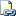# IIF (Transact-SQL)

Returns one of two values, depending on whether the Boolean expression evaluates to true or false in SQL Server 2012.Transact-SQL Syntax Conventions

## Syntax

``````IIF ( boolean_expression, true_value, false_value )
``````

## Arguments

• boolean_expression
A valid Boolean expression.

If this argument is not a Boolean expression, then a syntax error is raised.

• true_value
Value to return if boolean_expression evaluates to true.

• false_value
Value to return if boolean_expression evaluates to false.

## Return Types

Returns the data type with the highest precedence from the types in true_value and false_value. For more information, see Data Type Precedence (Transact-SQL).

## Remarks

IIF is a shorthand way for writing a CASE expression. It evaluates the Boolean expression passed as the first argument, and then returns either of the other two arguments based on the result of the evaluation. That is, the true_value is returned if the Boolean expression is true, and the false_value is returned if the Boolean expression is false or unknown. true_value and false_value can be of any type. The same rules that apply to the CASE expression for Boolean expressions, null handling, and return types also apply to IIF. For more information, see CASE (Transact-SQL).

The fact that IIF is translated into CASE also has an impact on other aspects of the behavior of this function. Since CASE expressions can be nested only up to the level of 10, IIF statements can also be nested only up to the maximum level of 10. Also, IIF is remoted to other servers as a semantically equivalent CASE expression, with all the behaviors of a remoted CASE expression.

## Examples

### A. Simple IIF example

``````DECLARE @a int = 45, @b int = 40;
SELECT IIF ( @a > @b, 'TRUE', 'FALSE' ) AS Result;
``````

Here is the result set.

``````Result
--------
TRUE

(1 row(s) affected)
``````

### B. IIF with NULL constants

``````SELECT IIF ( 45 > 30, NULL, NULL ) AS Result;
``````

The result of this statement is an error.

### C. IIF with NULL parameters

``````DECLARE @P INT = NULL, @S INT = NULL;
SELECT IIF ( 45 > 30, @p, @s ) AS Result;
``````

Here is the result set.

``````Result
--------
NULL

(1 row(s) affected)
``````